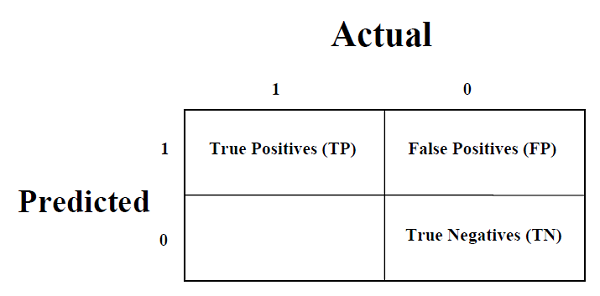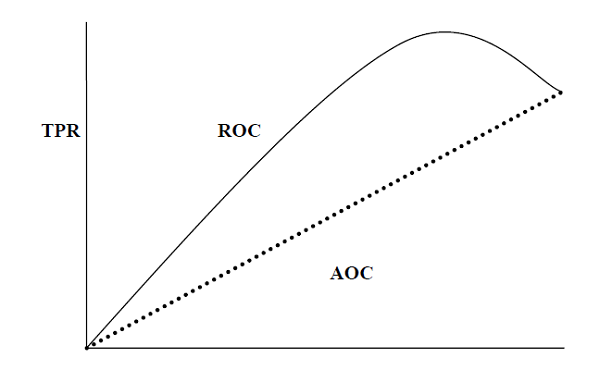# Machine Learning - Performance Metrics

There are various metrics which we can use to evaluate the performance of ML algorithms, classification as well as regression algorithms. We must carefully choose the metrics for evaluating ML performance because −

• How the performance of ML algorithms is measured and compared will be dependent entirely on the metric you choose.

• How you weight the importance of various characteristics in the result will be influenced completely by the metric you choose.

## Performance Metrics for Classification Problems

We have discussed classification and its algorithms in the previous chapters. Here, we are going to discuss various performance metrics that can be used to evaluate predictions for classification problems.

### Confusion Matrix

It is the easiest way to measure the performance of a classification problem where the output can be of two or more type of classes. A confusion matrix is nothing but a table with two dimensions viz. “Actual” and “Predicted” and furthermore, both the dimensions have “True Positives (TP)”, “True Negatives (TN)”, “False Positives (FP)”, “False Negatives (FN)” as shown below −Explanation of the terms associated with confusion matrix are as follows −

• True Positives (TP) − It is the case when both actual class & predicted class of data point is 1.

• True Negatives (TN) − It is the case when both actual class & predicted class of data point is 0.

• False Positives (FP) − It is the case when actual class of data point is 0 & predicted class of data point is 1.

• False Negatives (FN) − It is the case when actual class of data point is 1 & predicted class of data point is 0.

We can use confusion_matrix function of sklearn.metrics to compute Confusion Matrix of our classification model.

### Classification Accuracy

It is most common performance metric for classification algorithms. It may be defined as the number of correct predictions made as a ratio of all predictions made. We can easily calculate it by confusion matrix with the help of following formula −

$$Accuracy =\frac{TP+TN}{𝑇𝑃+𝐹𝑃+𝐹𝑁+𝑇𝑁}$$

We can use accuracy_score function of sklearn.metrics to compute accuracy of our classification model.

### Classification Report

This report consists of the scores of Precisions, Recall, F1 and Support. They are explained as follows −

### Precision

Precision, used in document retrievals, may be defined as the number of correct documents returned by our ML model. We can easily calculate it by confusion matrix with the help of following formula −

$$Precision=\frac{TP}{TP+FP}$$

### Recall or Sensitivity

Recall may be defined as the number of positives returned by our ML model. We can easily calculate it by confusion matrix with the help of following formula −

$$Recall =\frac{TP}{TP+FN}$$

### Specificity

Specificity, in contrast to recall, may be defined as the number of negatives returned by our ML model. We can easily calculate it by confusion matrix with the help of following formula −

$$Specificity =\frac{TN}{TN+FP}$$

### Support

Support may be defined as the number of samples of the true response that lies in each class of target values.

### F1 Score

This score will give us the harmonic mean of precision and recall. Mathematically, F1 score is the weighted average of the precision and recall. The best value of F1 would be 1 and worst would be 0. We can calculate F1 score with the help of following formula −

𝑭𝟏 = 𝟐 ∗ (𝒑𝒓𝒆𝒄𝒊𝒔𝒊𝒐𝒏 ∗ 𝒓𝒆𝒄𝒂𝒍𝒍) / (𝒑𝒓𝒆𝒄𝒊𝒔𝒊𝒐𝒏 + 𝒓𝒆𝒄𝒂𝒍𝒍)

F1 score is having equal relative contribution of precision and recall.

We can use classification_report function of sklearn.metrics to get the classification report of our classification model.

### AUC (Area Under ROC curve)

AUC (Area Under Curve)-ROC (Receiver Operating Characteristic) is a performance metric, based on varying threshold values, for classification problems. As name suggests, ROC is a probability curve and AUC measure the separability. In simple words, AUC-ROC metric will tell us about the capability of model in distinguishing the classes. Higher the AUC, better the model.

Mathematically, it can be created by plotting TPR (True Positive Rate) i.e. Sensitivity or recall vs FPR (False Positive Rate) i.e. 1-Specificity, at various threshold values. Following is the graph showing ROC, AUC having TPR at y-axis and FPR at x-axis −We can use roc_auc_score function of sklearn.metrics to compute AUC-ROC.

### LOGLOSS (Logarithmic Loss)

It is also called Logistic regression loss or cross-entropy loss. It basically defined on probability estimates and measures the performance of a classification model where the input is a probability value between 0 and 1. It can be understood more clearly by differentiating it with accuracy. As we know that accuracy is the count of predictions (predicted value = actual value) in our model whereas Log Loss is the amount of uncertainty of our prediction based on how much it varies from the actual label. With the help of Log Loss value, we can have more accurate view of the performance of our model. We can use log_loss function of sklearn.metrics to compute Log Loss.

### Example

The following is a simple recipe in Python which will give us an insight about how we can use the above explained performance metrics on binary classification model −

from sklearn.metrics import confusion_matrix
from sklearn.metrics import accuracy_score
from sklearn.metrics import classification_report
from sklearn.metrics import roc_auc_score
from sklearn.metrics import log_loss
X_actual = [1, 1, 0, 1, 0, 0, 1, 0, 0, 0]
Y_predic = [1, 0, 1, 1, 1, 0, 1, 1, 0, 0]
results = confusion_matrix(X_actual, Y_predic)
print ('Confusion Matrix :')
print(results)
print ('Accuracy Score is',accuracy_score(X_actual, Y_predic))
print ('Classification Report : ')
print (classification_report(X_actual, Y_predic))
print('AUC-ROC:',roc_auc_score(X_actual, Y_predic))
print('LOGLOSS Value is',log_loss(X_actual, Y_predic))


### Output

Confusion Matrix :
[
[3 3]
[1 3]
]
Accuracy Score is 0.6
Classification Report :
precision      recall      f1-score       support
0       0.75          0.50      0.60           6
1       0.50          0.75      0.60           4
micro avg     0.60          0.60      0.60           10
macro avg     0.62          0.62      0.60           10
weighted avg  0.65          0.60      0.60           10
AUC-ROC:  0.625
LOGLOSS Value is 13.815750437193334


## Performance Metrics for Regression Problems

We have discussed regression and its algorithms in previous chapters. Here, we are going to discuss various performance metrics that can be used to evaluate predictions for regression problems.

### Mean Absolute Error (MAE)

It is the simplest error metric used in regression problems. It is basically the sum of average of the absolute difference between the predicted and actual values. In simple words, with MAE, we can get an idea of how wrong the predictions were. MAE does not indicate the direction of the model i.e. no indication about underperformance or overperformance of the model. The following is the formula to calculate MAE −

$$MAE = \frac{1}{n}\sum|Y -\hat{Y}|$$

Here, 𝑌=Actual Output Values

And $\hat{Y}$= Predicted Output Values.

We can use mean_absolute_error function of sklearn.metrics to compute MAE.

### Mean Square Error (MSE)

MSE is like the MAE, but the only difference is that the it squares the difference of actual and predicted output values before summing them all instead of using the absolute value. The difference can be noticed in the following equation −

$$MSE = \frac{1}{n}\sum(Y -\hat{Y})$$

Here, 𝑌=Actual Output Values

And $\hat{Y}$ = Predicted Output Values.

We can use mean_squared_error function of sklearn.metrics to compute MSE.

### R Squared (R2)

R Squared metric is generally used for explanatory purpose and provides an indication of the goodness or fit of a set of predicted output values to the actual output values. The following formula will help us understanding it −

$$R^{2} = 1 -\frac{\frac{1}{n}\sum_{i{=1}}^n(Y_{i}-\hat{Y_{i}})^2}{\frac{1}{n}\sum_{i{=1}}^n(Y_{i}-\bar{Y_i)^2}}$$

In the above equation, numerator is MSE and the denominator is the variance in 𝑌 values.

We can use r2_score function of sklearn.metrics to compute R squared value.

### Example

The following is a simple recipe in Python which will give us an insight about how we can use the above explained performance metrics on regression model −

from sklearn.metrics import r2_score
from sklearn.metrics import mean_absolute_error
from sklearn.metrics import mean_squared_error
X_actual = [5, -1, 2, 10]
Y_predic = [3.5, -0.9, 2, 9.9]
print ('R Squared =',r2_score(X_actual, Y_predic))
print ('MAE =',mean_absolute_error(X_actual, Y_predic))
print ('MSE =',mean_squared_error(X_actual, Y_predic))


### Output

R Squared = 0.9656060606060606
MAE = 0.42499999999999993
MSE = 0.5674999999999999